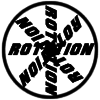#### You may also like### 8 Methods for Three by One

This problem in geometry has been solved in no less than EIGHT ways by a pair of students. How would you solve it? How many of their solutions can you follow? How are they the same or different? Which do you like best?### Rots and Refs

Follow hints using a little coordinate geometry, plane geometry and trig to see how matrices are used to work on transformations of the plane.### Reflect Again

Follow hints to investigate the matrix which gives a reflection of the plane in the line y=tanx. Show that the combination of two reflections in intersecting lines is a rotation.

# Matrix Meaning

##### Age 16 to 18Challenge Level

This problem involves the algebra of matrices and various geometric concepts associated with vectors and matrices. As you consider each point, make use of geometric or algebraic arguments as appropriate. If there is no definitive answer to a given part, try to give examples of when the question posed is or is not true.

In the five questions below: $R, S$ are rotation matrices; $P, Q$ are reflection matrices; $M,N$ are neither rotations nor reflections. Consider each part in 2D and 3D.

1. Is it always the case that $M+N = N + M$?
2. It it always the case that $RS= SR$?
3. It it always the case that $RP= PR$?
4. It it always the case that $PQ= QP$?
5. Is it ever the case that $MN = NM$?

How do the values of the determinants of the various matrices affect the results of these questions?